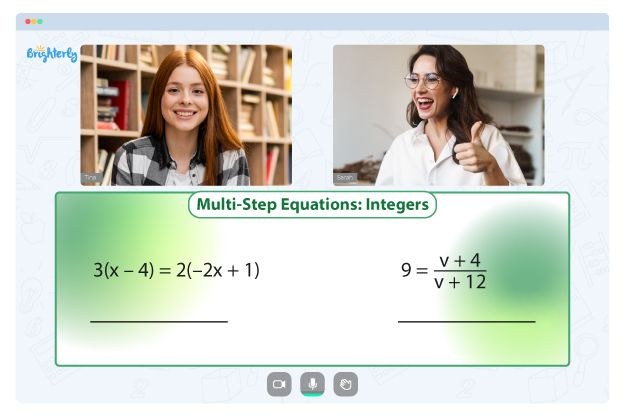# Least Common Denominator

Welcome to Brighterly’s comprehensive guide on the Least Common Denominator (LCD)! Here at Brighterly, we strive to make learning math a delightful and engaging experience for children of all ages. In this article, we’ll be unveiling the secrets of the least common denominator, guiding you through its intricacies, and showcasing its significance in various math problems, particularly those involving fractions. Together, we’ll unravel the mysteries of the LCD and uncover the joy of learning math!

## What is Least Common Denominator?

The Least Common Denominator (LCD) is the smallest multiple that two or more denominators of fractions share. It is used to compare, add, or subtract fractions with different denominators, making calculations easier and more manageable. In simple words, the LCD is the smallest number that all the denominators can divide evenly into.

## How to Find the Least Common Denominator?

1. List the multiples of each denominator.
2. Find the smallest multiple that all the denominators share. This is your LCD!

There are also more advanced methods, such as finding the Least Common Multiple (LCM) of the denominators or using the Prime Factorization method. For a more detailed explanation, check out this LCD tutorial by Math Is Fun.

## Ordering Fractions (Least to Greatest)

To order fractions from least to greatest, you need to first find the LCD of the given fractions’ denominators. Then, convert each fraction to an equivalent fraction with the LCD as the new denominator. Finally, compare the numerators and arrange the fractions accordingly. This method ensures that fractions are compared on a common scale, making it easy to determine their relative sizes.

## Application

The Least Common Denominator is commonly used in various real-life applications, such as:

• Sharing resources or dividing tasks among a group.
• Converting and comparing measurements in cooking, carpentry, or other activities.
• Simplifying complex math problems and making them easier to solve.

## Related Math Vocabulary

• Fraction: A numerical quantity that represents a part of a whole.
• Numerator: The top number in a fraction.
• Denominator: The bottom number in a fraction.
• Multiple: A number that can be divided by another number without a remainder.
• Least Common Multiple (LCM): The smallest multiple that two or more numbers have in common.

## Solved Examples on Least Common Denominator

Example 1: Find the LCD of 4 and 6.

Multiples of 4: 4, 8, 12, 16, … Multiples of 6: 6, 12, 18, 24, …

The smallest common multiple is 12, so the LCD is 12.

Example 2: Order the fractions 2/3, 1/2, and 3/4 from least to greatest.

Find the LCD of the denominators: 3, 2, and 4.

LCD = 12

Convert the fractions to equivalent fractions with the LCD as the denominator:

2/3 = 8/12, 1/2 = 6/12, and 3/4 = 9/12

Order the fractions: 1/2 (6/12), 2/3 (8/12), and 3/4 (9/12)

## Practice Questions on Least Common Denominator

1. Find the LCD of 5 and 10.
2. Find the LCD of 8 and 12.
3. Order the fractions 3/5, 2/3, and 5/6 from least to greatest.

## Conclusion

At Brighterly, we’re passionate about making math accessible and enjoyable for everyone. As we’ve explored in this guide, understanding the Least Common Denominator is crucial for working with fractions, as it simplifies calculations and assists in comparing or ordering fractions effectively. This essential concept not only aids in solving math problems but also has a multitude of real-life applications that you’ll encounter in everyday situations.

By embracing the Brighterly approach, you’ll develop a solid foundation in math concepts like the Least Common Denominator, empowering you to tackle challenges with confidence and enthusiasm. Through practice, persistence, and the support of our engaging learning materials, you’ll soon become proficient in finding the LCD and applying it in various contexts, both in and out of the classroom.

Remember, the journey to math mastery is an ongoing adventure. As you continue to explore the exciting world of mathematics with Brighterly by your side, you’ll unlock new skills, gain deeper insights, and uncover the true joy of learning. So, keep practicing, stay curious, and never stop growing!

## Frequently Asked Questions on Least Common Denominator

### Can the LCD be one of the original denominators?

Yes, the LCD can be one of the original denominators if it’s a multiple of the other denominators. For example, the LCD of 3 and 6 is 6, which is one of the original denominators.

### Can there be more than one LCD for a set of fractions?

No, there can only be one LCD for a set of fractions. The LCD is the smallest common multiple of the denominators, and there cannot be multiple smallest common multiples.

### Do you need to find the LCD when multiplying or dividing fractions?

No, you don’t need to find the LCD when multiplying or dividing fractions. You can directly multiply or divide the numerators and denominators of the fractions.

### How do I find the LCD of more than two denominators?

To find the LCD of more than two denominators, list the multiples of each denominator, and find the smallest multiple that they all share. Alternatively, you can use the Least Common Multiple (LCM) or the Prime Factorization method to find the LCD.

Information Sources

Keep practicing and exploring the world of math with Brighterly! We’re here to make math fun and engaging for all children. For more resources and guides, visit our website, and don’t forget to share your math adventures with your friends!

Problems with Equations?• Is your child finding it hard to grasp equations fundamentals?
• An online tutor could be of assistance.

Does your child struggle with mastering the basics of equations? Try lessons with an online tutor.